`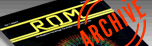ROM MAGAZINE ISSUE 7 — AUGUST/SEPTEMBER 1984 / PAGE 44`

 ALPHAMINER by Tom Tuong Tran     The Atari computer is the easiest computer with which to access graphics in Basic without having to use any machine language. In each game I write I try to have some educational content. It may be fun to play but, at the same time, should teach something at an elementary level. In issue number 5, I did a shoot'em up type game, also taught basic mathematics. The current game Alphaminer teaches simple spelling.     The game begins with a title screen showing a lady singing the Alphabet song. As each letter or word appears on the screen the lady moves her mouth, thus appearing to be singing.     To the right of the lady is a menu showing four choices: 1) Alphabetical (A-Z), 2) Reverse (Z-A), 3) Any order, and 4) Quit. Below is shown the high score in the form HI CASH = \$. Push ` 1 ' on the keyboard to begin play. A little man will appear at the top left hand corner of the screen. Letters will be randomly displayed on the screen below and then covered up with different coloured earth.     Because you pushed the key, the player must dig up the letters in the correct order form A-Z. So try to remember where the letters were scattered. Now move your man with the joystick to the right through the bank's door. The cash (points) will now go up to \$2500. Now move the man to the right onto the purple car. The car will then move automatically and place the man on the elevator. To lower the elevator move the joystick down. When you wish to stop at a certain level, pushed the fire button. After you have pushed the button the elevator will stop, allowing your man to get off. He can then move through the earth, mining for letters. He must find the letter `A' first since we close the 1 selection on the menu. If we had chosen 2 we would have to get `2' first. If we had picked 3 we could get anyone that we wanted first.     Rocks block the miner's path, making him go around them. If you can't remember where the letter `A' is, push `H' for help. The `A' will now appear, making it easily recoverable. The miner can move in any of the eight joystick positions just by pushing the joystick in the direction desired. When moving up, your miner will build a ladder. He moves to the right or left and if there is no earth there he will die and the game will be over. So be careful when going up and down ladders. Another way of dying is to move outside the earth without having the elevator beside you.     The more moves that you make to find a letter, the lower your score will be after you recover it. When moving up you lose 35 points a step, moving left and right through earth you lose 20 dollars, and left and right through empty space, 10 dollars. After you touch the key that corresponds to the letter on the screen, move the miner back to the elevator and push your button. This will take you back up to the surface. Push your button again when you get to the top and move back onto the car. This will take you back to the bank where you can pick up your loot for recovering the letters. Try not to use the Help key to find the letters, so that the game becomes more challenging.     By redefining the character set, I was able to make the graphics look more interesting. Instead of having a block for a man, a realistic looking man was created. Also, by using control characters (Atari's built in character set), I was able to cut out a lot of work redefining the characters myself. I hope that you will enjoy this game.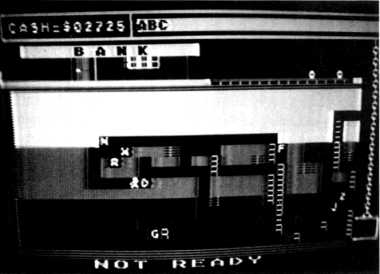1 REM ---ROM MAGAZINE--------- 2 REM WRITTEN BY:Tom Tuong Tran 3 REM -ROM EDUCATION SOFTWARE-- 10 DIM P1\$(20),CAR\$(15),ELE\$(25),A(100),T(100),K(4),C\$(5):N1=1:N0=0:N256=256:N23=23:N11=11:N7=7:N36=36:N12=12 20 N76=76:N22=22:N2=2:N14=14:N59=59:N33=33:N10=10:N5=5:N37=37:N13=13:N5=5:N40=40:N9=9:N6=6:N76=76:N4=4:N202=202 30 N128=128:N3=3:WAIT=7000:LINE=5000:WAIT1=8000:N53761=53761 50 N15=15:N82=82:Nl9=19:x(1)=65:K(2)=193:K(3)=97:K(4)=225:GOTO 1000 60 G=CASH:CI=N0 70 IF G>0.99 THEN G=G/N10:Cl=C1+N1:GOTO 70 80 POSITION 12-Cl,Nl:? CASH:RETURN 100 SOUND N0,120,N10,N10 105 IF (AL=L OR Z=N0) AND NOT FLAG THEN 160 110 IF H=26 THEN 841 140 POSITION N0,N23:? "    NOT READY "; 150 MOVE=V:X=X2:Y=Y2:SOUND N0,N0,N0,N0:GOTO 210 160 SOUND 0,200,N0,N0:AL=AL+Z:FLAG=Nl:H=H+Nl:AL1=TABLE+H:AL2=L:POKE AL1,AL2:IF KEY=255 THEN POKE MOVE,N0:GOTO 150 165 POKE SC+40*(Y+DY)+(X+DX),N0:POKE 764,255:GOTO 150 200 IF Y1=N3 THEN Y=N5:X=N37:GOTO 510 205 Y=Yl+N2:X=N37:MOVE=SC+40*Y+X:R=N1 210 ST=STICK(N0):IF ST<>Nl5 THEN POKE N53761,N59:GOTO 330 215 IF Y=Y1+N2 AND X=N37 AND STRIG(N0)=N0 THEN 850 216 IF R=N0 THEN POSITION N0,N23:? "YOU ARE FALLING";:GOTO 800 217 IF CASH<=N0 THEN GOTO 840 218 GOSUB 60:KEY=PEEK(764):IF KEY=57 THEN 220 219 GOTO 210 220 FOR W=N1 TO N:IF AL=T(W) THEN POKE A(W),T(W) 230 NEXT W:GOTO 210 330 DY=(ST=Nl3 OR ST=N9 OR ST=N5)-(ST=Nl4 OR ST=N10 OR ST=N6) 340 DX=(ST=N7 OR ST=N6 OR ST=N5)-(ST=Nll OR ST=N10 OR ST=N9):CH=N1 350 X2=X:Y2=Y:X=X+DX:Y=Y+DY:V=MOVE:MOVE=SC+40*Y+X:U=L:L=PEEK(MOVE):R=PEEK(MOVE+N40):O=N0:CASH=CASH-10 351 IF X=N0 OR X=38 OR Y=N6 OR Y=22 OR L=77 OR L=78 OR L=79 THEN MOVE=V:X=X2:Y=Y2:R=N1 357 FOR W=N1 TO N:IF MOVE=A(W) THEN POKE A(W),T(W):MOVE=V:X=X2:Y=Y2:A(W)=N0 358 NEXT W:POKE N53761,N0 359 IF ST=N14 THEN O=N6:CASH=CASH-N5 360 IF U=6 THEN O=U 365 IF L=N202 THEN POSITION N0,N23:? " HARD ROCK ";:MOVE=V:X=X2:Y=Y2 370 IF L<59 AND L>32 THEN 100 375 IF L<>N0 THEN CASH=CASH-20 380 POKE V,O:POKE MOVE,N76:GOTO 210 400 IF ST=Nll THEN DX=-Nl 410 IF ST=N7 THEN DX=N1 420 POKE P,Pl:IF ST=N15 THEN POKE P,N0 430 X=X+DX:P=SC+40*Y+X:PI=PEEK(P):IF PEEK(P+40)=85 THEN X=X-DX:P=SC+40*Y+X 440 POKE P,N76:RETURN 500 X=N2:Y=N5:P=SC+40*Y+X:POKE P, N76:Pl=N0:FLAG=1 510 ST=STICK(N0):IF ST<>Nl5 THEN GOSUB 400 522 IF X=N0 THEN X=X+1 523 IF X=38 THEN X=X-1 524 IF X=6 AND FLAG THEN GOSUB 600 525 IF X=37 AND NOT FLAG THEN 850 526 IF X=19 AND NOT FLAG THEN E1=Nl:E2=N10:E3=NI:GOSUB 910 527 IF X=35 AND FLAG THEN E1=N10:E2=Nl:E3=-Nl:GOSUB 910 590 GOTO 510 600 POKE P,PI:FOR K=N7 TO 8:POSITION K,N4:? "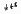";:GOSUB WAIT:? "←":NEXT K 601 IF CASH=N0 THEN I=250:GOTO 603 602 1=200:POKE AL1,AL2+128 603 FOR K=1 TO N10:SOUND N0,K+N15,8,NlO:CASH=CASH+I:GOSUB 60:POKE 77 ,N0 610 SOUND N0,N0,N0,N0:NEXT K:POSITION N7,N4:? "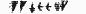":FLAG=N0:X=8:GOSUB 400:RETURN 700 FOR K=N6 TO N7:POSITION K,N5:? " ↓← ":GOSUB 7000:NEXT K:POSITION N6,N5:? "":FLAG=N0:RETURN 710 FOR PO=0 TO 150:SOUND 0,PO,10,PO/15+2:NEXT PO 720 FOR PO=0 TO 240 STEP 5:VOL=14-PO/20:SOUND VO,PO,O,VOL:SOUND Vl,P0,8,VOL 730 SOUND V2,PO+15,2,VOL:NEXT P0:GOSUB 54:RETURN 800 DOWN=MOVE:S=N0 810 POKE DOWN,N0:DOWN=DOWN+N40:IF PEEK(DOWN)<>N0 THEN POKE DOWN-N40,91:GOTO 840 820 POKE DOWN,127:S=S+NIO:FOR Q=S TO S+10:SOUND O,Q,10,17-Q/l0:NEXT Q:GOTO 810 840 FOR S=1 TO 15:SOUND 0,120,8,15-S:NEXT S:SOUND 0,0,0,0 841 POSITION N0,N23:? "    GAME - OVER";:IF HCASHN3 AND FLAG THEN DY=-N1 860 POSITION N36,Yl:? "   ↓←←← ↓←←←   ":Yl=Yl+DY:GOSUB 900:GOSUB WAIT1 861 IF STRIG(N0)=N0 THEN GOSUB WAIT:GOTO 200 865 IF Yl>=18 THEN DY=-N1 866 IF Yl=N3 THEN DY=N1 870 GOTO 860 900 POSITION N36,Yl:? ELE\$:RETURN 910 POKE P,N0:CAR\$(Nl,Nl)=CHR\$(12):DX=NI:IF X=35 THEN DX=-N1 915 FOR K=E1 TO E2 STEP E3:GOSUB 950:GOSUB WAIT1 920 POSITION X1,4:? "           ":X1=X1+DX:NEXT K:CAR\$(Nl,Nl)=CHR\$(32):GOSUB 950:X=35:IF E3=-N1 THEN X=19 930 ST=NI5:GOSUB 400:RETURN 950 POSITION Xl,N4:? CAR\$:RETURN 1000 REM MACHINE LANGUAGE SUB. TO MOVE CHARACTER SET 1001 CH=PEEK(106)-8:CHSET=CH*256:POKE 204,CH:POKE 206,224 1002 FOR K=1 TO 20:READ B:Pl\$(K,K=CHR\$(B):NEXT K:K=USR(ADR(Pl\$)) 1003 DATA 104,162,4,160,0,177,205,145,203,200,208,249,230,206,230,204,202,208,242,96 1004 READ A:IF A=-1 THEN 2000 1005 FOR J=0 TO 7:READ B:POKE CHSET+A*8+J,B:NEXT J 1006 GOTO 1004 1008 DATA 3,40,40,16,16,16,40,170,170 1009 DATA 5,0,0,0,0,7,31,120,0 1010 DATA 6,126,66,66,66,126,66,66,66 1011 DATA 10,0,31,48,106,202,248,15,0 1012 DATA 11,0,192,96,48,49,97,193,1 1013 DATA 12,0,3,6,12,140,134,131,128 1014 DATA 13,0,0,0,0,126,0,0,0 1015 DATA 27,0,0,0,0,128,224,56,0 1016 DATA 28,5,5,5,13,9,49,73,133 1017 DATA 29,0,0,0,126,0,0,126,0 1018 DATA 30,78,49,0,0,0,0,0,0 1019 DATA 59,20,21,81,66,82,20,5,1 1020 DATA 60,20,84,69,129,133,20,80,64 1021 DATA 61,0,1,5,16,85,5,0,0 1022 DATA 62,0,64,80,4,85,80,0,0 1023 DATA 63,34,220,0,0,0,0,0,0 1024 DATA 64,117,85,85,85,85,85,85,85 1025 DATA 72,170,170,170,170,170,170,170,170 1026 DATA 73,213,213,213,255,213,213,213,255 1027 DATA 74,170,85,170,85,170,85,170,85 1028 DATA 75,0,248,12,166,163,14,248,0 1029 DATA 76,60,60,27,126,216,60,102,102 1030 DATA 79,24,24,60,102,195,195,255,255 1031 DATA 80,255,255,255,255,85,170,85,170 1032 DATA 83,170,170,170,170,0,0,0,0 1033 DATA 84,170,170,128,60,126,102,126,60 1034 DATA 85,85,85,161,161,85,170,85,170 1035 DATA 89,160,160,160,176,144,140,146,161 1036 DATA 91,60,126,102,231,129,231,231,231 1037 DATA 96,208,212,213,212,208,192,192,192 1038 DATA 123,24,24,60,110,102,118,60,24 1039 DATA 127,102,102,60,24,126,219,60,60 1040 DATA -1 2000 GRAPHICS 0 2010 LBYTE=PEEK(560):HBYTE=PEEK(561):SCREEN=LBYTE+HBYTE*256+4 2020 POKE SCREEN-1,64+7 2030 FOR K=1 TO 2:POKE SCREEN+K+1,6:NEXT K 2040 FOR K=1 TO 14:POKE SCREEN+K+3,4:NEXT K 2050 FOR K=1 TO 2:POKE SCREEN+K+17,7:NEXT K 2060 POKE SCREEN+21,65:POKE SCREEN+22,LBYTE:POKE SCREEN+23,HBYTE 2500 SC=PEEK(88)+PEEK(89)*N256:SETCOLOR N2,N0,NIO:POKE 756,CH:POKE 752,N1:CAR\$="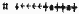":TABLE=SC+52:H=0 2510 ELE\$="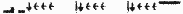":Y1=3:X1=20:POKE 82,N0:POSITION N5,N0:CASH=N0 2520 ? "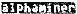":? "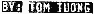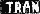":? "↓↓ 1)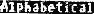(A-Z)" 2530 ? " ↓2)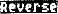(Z-A)":? "↓3)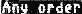(random)":? " ↓4)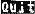":? "↓↓CHOOSE ONE ?" 2540 POSITION N23,N3 2541 ? "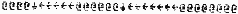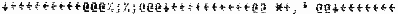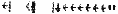"; 2542 ? "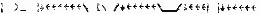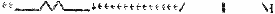I  CASH=\$";HCASH 2600 RESTORE 6000:J1=N3:J2=16:C=N0:M=M+NI:IF M>N4 THEN M=N1 2610 READ A,B:IF A=N0 THEN POSITION 29,N10:? "]^":GOSUB WAIT:GOTO 2692 2611 GOSUB 2612:GOTO 2610 2612 POSITION 29,N10:? "[/":POKE 540,B:SOUND N0,A,N10,N10:POSITION J1,J2:? CHR\$(K(M)+C);:C=C+1:J1=J1+N1 2615 IF J1>N15 THEN J1=N3:POSITION 3,16:? "         " 2620 IF PEEK(540)<>N0 THEN 2650 2630 POSITION 29,N10:? "]^":SOUND N0,N0,N0,N0:FOR K=1 TO 10:NEXT K:RETURN 2650 J=PEEK(764):IF J=31 THEN AL=N33:Z=NI:GOTO 2700 2660 IF J=30 THEN AL=58:Z=-N1:GOTO 2700 2680 IF J=26 THEN Z=N0:GOTO 2700 2690 IF J=24 THEN RUN "D:MENU.*" 2691 GOTO 2620 2692 READ A,B,C\$:IF A=N0 THEN 2695 2693 POSITION 29,N10:? "[\":SOUND N0,A,N10,N10:POKE 540,B:POSITION (20-LEN(C\$))/2,16 2694 ? C\$:GOSUB 2620:POSITION 5,16:? "    ":GOTO 2692 2695 FOR K=1 TO 100:NEXT K:POSITION 30,7:? 11%;":GOSUB WAIT:POSITION 30,7:? ",":FOR K=1 TO 300:NEXT K:GOTO 2600 2700 GRAPHICS N0:POKE 756,CH:SETCOLOR N2,N0,NIO:POKE SCREEN-1,64+2:FOR K=1 TO 22:POKE SCREEN+K+1,2:NEXT K 2710 FOR K=1 TO 1:POKE SCREEN+K+23,6:NEXT K:POKE 82,N0:SOUND N0,N0,N0,N0:POKE 752,N1:POKE 764,255 3000 ? "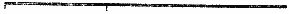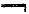";:? "|CASH=\$00000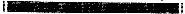" 3010 ? "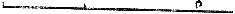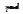";:? "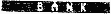":? "        " 3015 ? "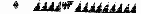" 3020 ? "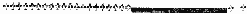";:FOR K=1 TO 16:? "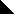";:NEXT K 3030 POSITION N0,N22:? "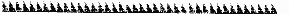";:GOSUB-900:GOSUB 950:K=N33 3032 FOR W=N10 TO N0 STEP -N1:SETCOLOR N2,N0,W:FOR Q=1 TO 10:NEXT Q:NEXT W 3035 POSITION O,N23:? "SET UP....";K-32;:IF K=59 THEN 3055 3040 X=NI+INT(RND(N0)*35):Y=N7+INT(RND(N0)*N15):A=SC+N40*Y+X:IF PEEK(A)<>N0 THEN 3040 3045 IF INT(RND(N0)*2)=N0 THEN POKE A,K:K=K+NI:GOTO 3035 3050 POKE A,N202:GOTO 3040 3055 N=N0:Q=N7:B=N128:GOSUB 3060:Q=N12:B=200:GOSUB 3060:Q=17:B=72:GOSUB 3060:GOTO 500 3060 FOR Y=Q TO Q+N4:FOR X=N1 TO 35:A=SC+40*Y+X:T=PEEK(A):IF T<>N0 THEN N=N+N1:A(N)=A:T(N)=T 3070 POKE A,B:NEXT X:NEXT Y:RETURN 4000 GOTO 4000 6000 DATA 121,20,121,20,81,20,81,20,72,20,72,20,81,40,91,20,91,20,96,20,96,20,108,10,108,10,108,10,108,10 6010 DATA 121,40,81,20,81,20,91,40,96,20,96,20,108,40,81,40,91,40,96,40,108,20,0,250 6020 DATA 121,20,NOW,121,20,I,81,20,KNOW,81,20,MY,72,20,A,72,20,B,81 ,40,C'S 6030 DATA 91,20,NEXT,91,20,TIME,96,20,WON'T,96,20,YOU,108,20,SING,108,20,WITH,121,40,ME,0,0,0 7000 FOR Q=N1 TO 40:NEXT Q:RETURN 7010 IF L=67 THEN FOR Q=10 TO 70:SOUND 0,Q,14,7-Q/10:NEXT Q:FOR S=1 TO 10:SOUND 0,120,8,10-S:NEXT S 8000 FOR Q=N1 TO 30:NEXT Q:RETURN 9000 7 PEEK(764):GOTO 9000 D 0,Q,14,7-Q/10:NEXT Q:FOR S=1 TO 10:SOUND 0,120,8K DATA CHECK 0 REM * DATA CHECK :ALPHAMINER * 1 DATA 7492,520,629,312,55,578,671,876,69,625,393,512,753,269,560,156,243,271 200 DATA 9619,428,501,680,167,38,918,897,835,96,324,960,297,891,517,944,688,438 360 DATA 9195,307,562,788,990,371,670,583,518,789,555,234,787,486,470,210,159,716 527 DATA 8784,455,840,699,195,635,518,609,540,11,770,284,351,234,946,523,514,660 850 DATA 9253,731,478,949,648,566,849,339,755,173,340,943,290,556,506,28,323,779 1005 DATA 9008,237,928,18,650,55,8,997,6,640,811,841,755,662,882,82,663,773 1023 DATA 5453,695,74,374,372,206,87,177,244,300,958,276,206,387,319,391,164,223 1040 DATA 8129,792,54,53,508,742,794,800,284,391,220,0,276,403,658,860,771,523 2611 DATA 7730,612,376,263,911,788,219,465,57,43,944,102,196,316,882,743,62,751 3010 DATA 8685,186,951,205,707,838,550,262,527,659,287,845,464,924,310,315,212,443 7000 DATA 1320,305,88,305,622